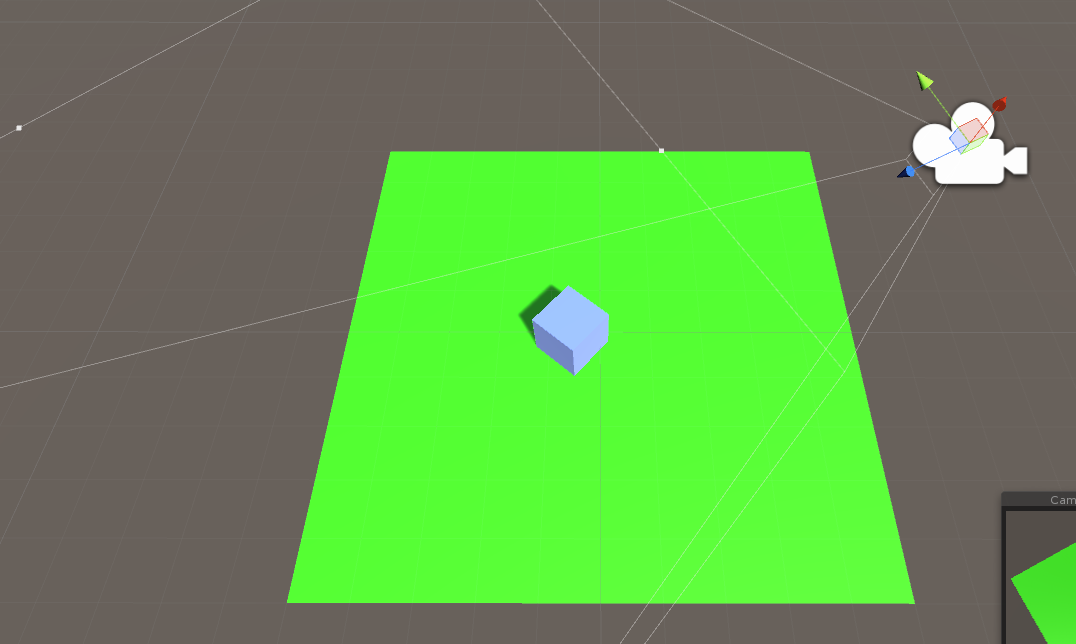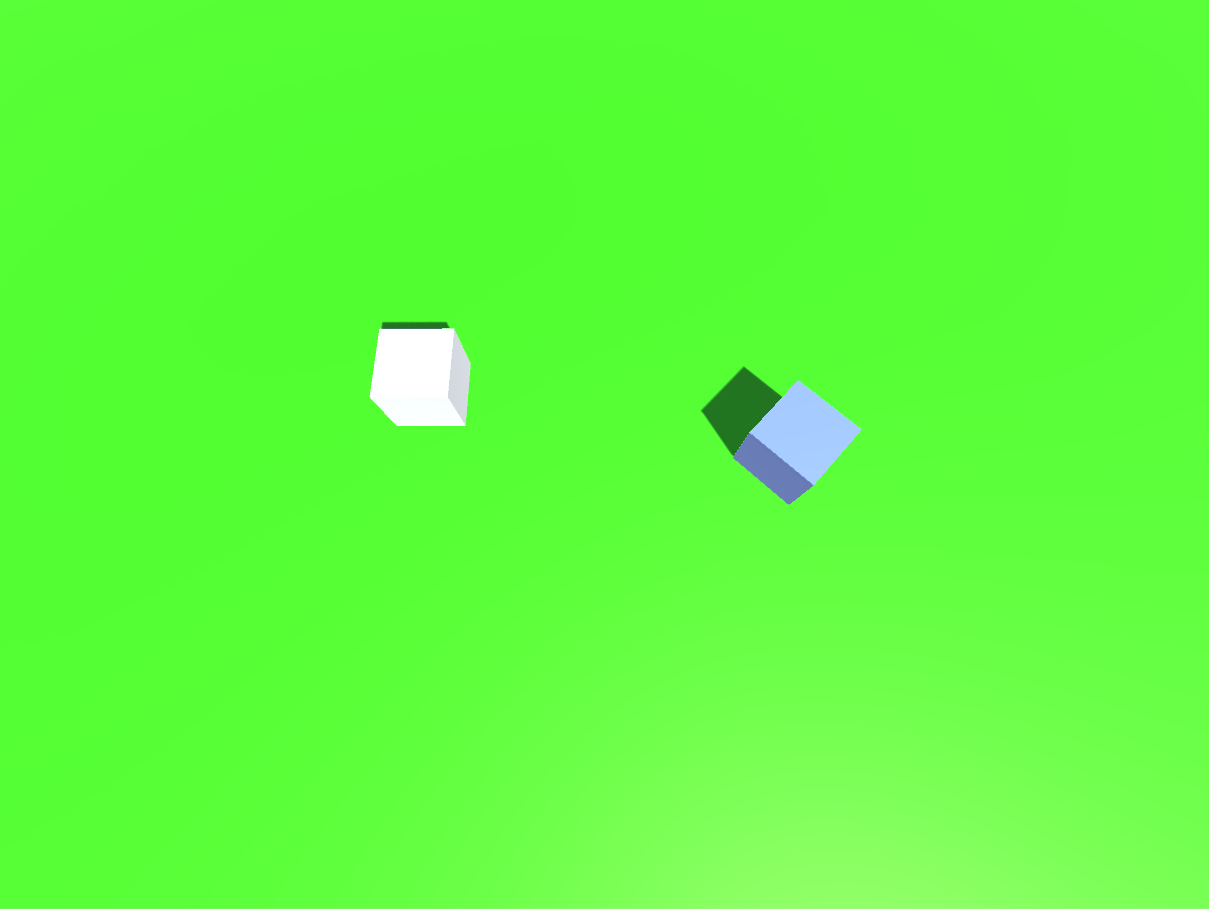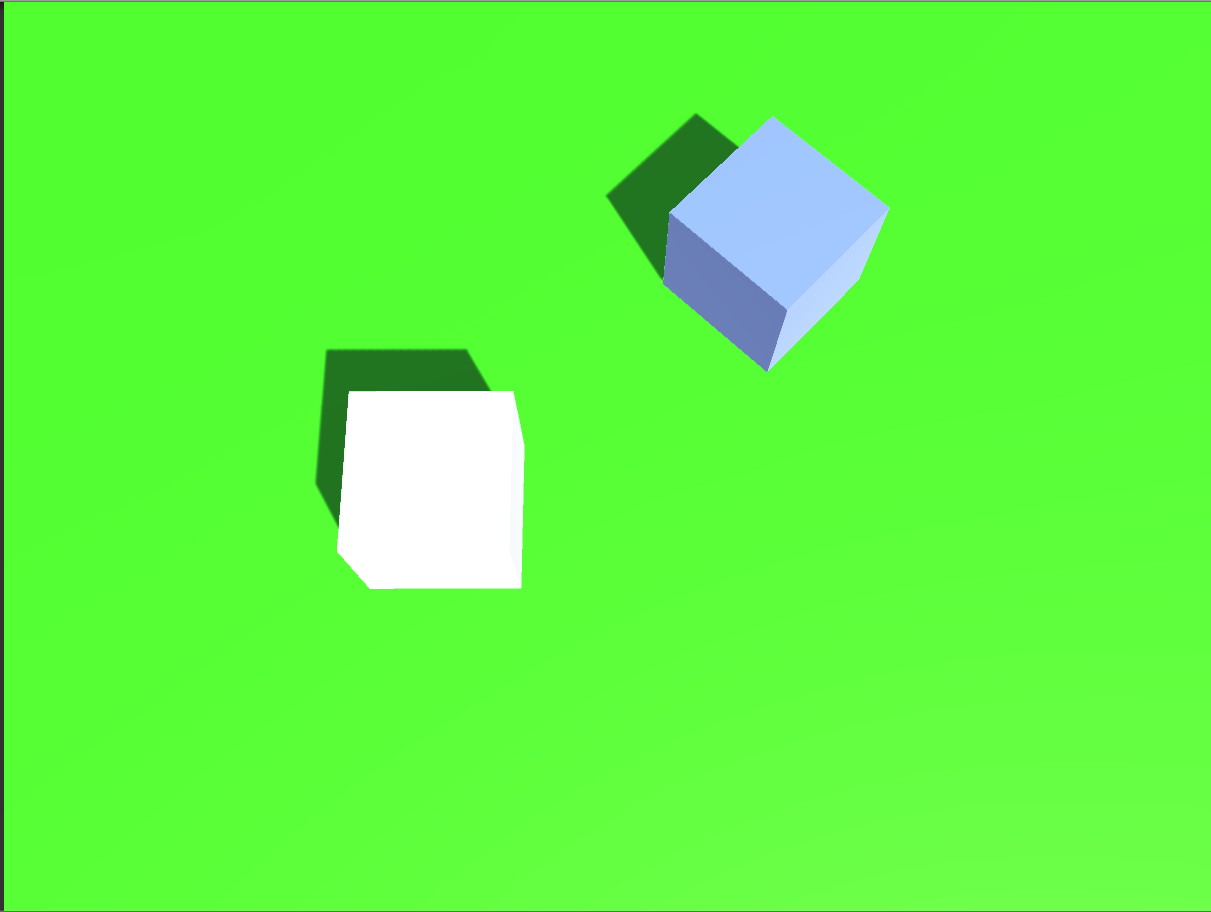2017-06-08 21:41:27 u014805591 阅读数 1564
• ###### Unity3D着色器程序设计-CG版

本课程将带领大家学习Unity3D中的着色器程序

21391 人正在学习 去看看 杨振``````using UnityEngine;
using System.Collections;

public class MouseOrbit : MonoBehaviour {

/// <summary>
/// 得到物体的名字
/// </summary>
//public string Getobejctname;

public Transform initPosObject;

//public Transform targetObject;

/// <summary>
/// ---------------限制速度-----------------------
/// </summary>

public float xSpeed = 350.0f;
public float ySpeed = 250.0f;

/// <summary>
/// ------------ 限制X和Y的角度-------------------
/// </summary>

public float xMinLimit = -20.0f;
public float xMaxLimit = 80.0f;

public float yMinLimit = -20.0f;
public float yMaxLimit = 80.0f;

/// <summary>
/// ---------------限制距离-----------------------
/// </summary>

public float initDis = 2.0f;
public float minDis = 2.0f;
public float maxDis = 10.0f;

/// <summary>
/// ------------------鼠标滚轮速度------------------
/// </summary>

public float wheelSpeed = 5.0f;

public float yMoveSpeed = 1.0f;
public float xMoveSpeed = 1.0f;

public  float x = 130.0f;

public  float y = 30.0f;

private float distance = 0.0f;

public bool IsOnHove = false ;

// Use this for initialization
void Start ()
{
InitOrbitCamera(initPosObject);
}

// public Transform getOb;
bool zoomFlag = false;
// Update is called once per frame
void Update ()
{

//鼠标浮在NGUI滑条上
if (!IsOnHove)
{
//距离相比较
distance = Vector3.Distance(Vector3.zero,transform.localPosition);

x += Input.GetAxis("Mouse X") * xSpeed * 0.02f; // 鼠标横向输入
x = ClampAngle(x, xMinLimit, xMaxLimit);
y += Input.GetAxis("Mouse Y") * ySpeed * -0.02f; //鼠标纵向输入
y = ClampAngle(y, yMinLimit, yMaxLimit);//得到一个适中 的角度

if (Input.GetMouseButton(0))
{
x += Input.GetAxis("Mouse X") * xSpeed * 0.02f; // 鼠标横向输入
x = ClampAngle(x, xMinLimit, xMaxLimit);
y += Input.GetAxis("Mouse Y") * ySpeed * -0.02f; //鼠标纵向输入
y = ClampAngle(y, yMinLimit, yMaxLimit);//得到一个适中 的角度
}
if (Input.GetMouseButtonDown(1))
{
if (!zoomFlag)
{
this.GetComponent<Camera>().fieldOfView = 12;
}
else {
this.GetComponent<Camera>().fieldOfView = 50;
}
zoomFlag = !zoomFlag;

}

distance-= Input.GetAxis("Mouse ScrollWheel")*wheelSpeed;//距离是用滚轮控制
}
//得到适中的距离
distance = Mathf.Clamp(distance,minDis,maxDis);
//得到旋转的角度
if (moveflag)
{
Quaternion localRotation = Quaternion.Euler(y, 0, 0);

Vector3 localPosition = localRotation * new Vector3(0.0f, 0.0f, -1*distance) + Vector3.zero;

transform.localRotation = localRotation;
transform.localPosition = localPosition;

Quaternion rotationP = Quaternion.Euler(0,x, 0);

transform.parent.rotation = rotationP;

if(Mathf.Abs(Input.GetAxis("Horizontal"))>0||Mathf.Abs(Input.GetAxis("Vertical"))>0)
{
transform.parent.Translate(new Vector3(Input.GetAxis("Horizontal")*xMoveSpeed, 0, Input.GetAxis("Vertical")*xMoveSpeed)*Time.deltaTime);
}
if(Input.GetMouseButton(2))
{
transform.parent.transform.Translate(0,Input.GetAxis("Mouse Y") * yMoveSpeed,0);
}
}

}
/// <summary>
/// 设置相机以某个物体为中心
/// </summary>
/// <param name="cObj"></param>
public void InitOrbitCamera(Transform cObj)
{
if(cObj!=null)
{
transform.parent.position = cObj.position;
}
else
{
transform.parent.position = Vector3.zero;
}

transform.localRotation = Quaternion.Euler(y,x,0);
transform.localPosition = Quaternion.Euler(y,x,0) * new Vector3(0.0f,0.0f,-1*initDis);
}
//限制角度
float ClampAngle(float angle,float min,float max)
{
if (angle < -360.0f)
{
angle += 360.0f;
}
if (angle > 360.0f)
{
angle -= 360.0f;
}
return Mathf.Clamp (angle, min, max);
}

private Vector3 targetPos;
private bool moveflag = true;
private bool backFlag = false;

/// <summary>
/// 返回目标点
/// </summary>
/// <param name="obj"></param>
/// <returns></returns>
public IEnumerator BackStartPos(Transform obj)
{
backFlag = true;
yield return 1;
StartCoroutine(SetCameraTarget(obj));
yield return 1;
}

public float TempY;

public float TempX;
/// <summary>
/// 设置相机的目标点
/// </summary>
/// <param name="obj"></param>
/// <returns></returns>
public IEnumerator SetCameraTarget(Transform obj)
{
TempY = y;

TempX = x;

bool isMove = true;
moveflag = false;

Quaternion localRotation = Quaternion.Euler(y, 0, 0);

Quaternion rotationP = Quaternion.Euler(0, x, 0);

Vector3 camEndPos = localRotation * new Vector3(0.0f, 0.0f, -1 * initDis);
Vector3 camPos;

if (obj.transform.GetComponent<Collider>())
{
camPos = obj.transform.GetComponent<Collider>().bounds.center;
}
else
{
camPos = obj.transform.position;
}
while (isMove)
{

transform.localRotation = Quaternion.Lerp(transform.localRotation, localRotation,0.05f);
transform.localPosition = Vector3.Lerp(transform.localPosition, camEndPos, 0.05f);
transform.parent.position = Vector3.Lerp(transform.parent.position, camPos, 0.05f);

transform.parent.rotation = Quaternion.Lerp(transform.parent.rotation, rotationP, 0.05f);

yield return new WaitForSeconds(0.01f);

if ((Vector3.Distance(transform.parent.position, camPos) < 0.005f) && (Mathf.Abs((Vector3.Distance(transform.localPosition, Vector3.zero)) - initDis) < 0.01f))
{
isMove = false;

//恢复输入的y的值
y = TempY;
//恢复输入的x的值
x = TempX;

moveflag = true;
transform.parent.position = camPos;
backFlag = false;

IsOnHove = false;
break;
}

}
}
}
``````unity3d中的摄像机 相关内容

2013-08-21 18:37:07 kfqcome 阅读数 32572
• ###### Unity3D着色器程序设计-CG版

本课程将带领大家学习Unity3D中的着色器程序

21391 人正在学习 去看看 杨振

# 二 属性

## 2 Background## 4 Projection## 8 Normalized viewport rect

X：摄像机视图在屏幕上被绘制的水平初始位置

Y：摄像机视图在屏幕上被绘制的垂直初始位置

W：摄像机视图输出图像占屏幕宽度的比例

H：摄像机视图输出图像占屏幕高度的比例

U3D屏幕的坐标系是以左下角为坐标原点，向右为X轴，向上为Y轴

## 10 Rendering Path

• Use Player Settings，摄像机会使用在玩家配置里面指定的渲染路径

• Vertex Lit，所有被该摄像机渲染的对象都会被渲染成Vertex-Lit对象

• Forward，所有的对象都会被渲染为一个材质对应一个通道

• Deferred Lighting，所有的对象在没有光照的情况下绘制一次，然后在渲染队列的末端将所有对象的光照一起绘制，也就是所谓的“延迟光照”技术。

# 三 示例

• 创建一个新的摄像机，取名为”Background Camera”

• 创建一个新的GUI贴图，取名为”Background Image”

• 单击Background Image的监视器面板的”Layer”下拉按钮，创建一个新的层，取名为”Background Image”

• 设置好贴图Background Image的大小和位置，同时将它的层设定为“Background Image”

• 选中Background Camera，对它的一些属性进行这样的操作：取消勾选Flare Layer和Audio Listener，设定清除标志为Solid Color，设定深度为-1，先将Culling Mask设为nothing，然后设为Background Image

backgroundGUITexture.pixelInset.width = Screen.width;

backgroundGUITexture.pixelInset.height = Screen.height;

backgroundGUITexture.pixelInset.x = 0;

backgroundGUITexture.pixelInset.y = 0;

backgroundGUITexture.pixelInset.width = Screen.currentResolution.width;

backgroundGUITexture.pixelInset.height = Screen.currentResolution.height;

unity3d中的摄像机 相关内容

2016-02-19 11:08:49 wuyt2008 阅读数 6221
• ###### Unity3D着色器程序设计-CG版

本课程将带领大家学习Unity3D中的着色器程序

21391 人正在学习 去看看 杨振unity3d中的摄像机 相关内容

2019-01-15 11:14:26 wl1611102 阅读数 392
• ###### Unity3D着色器程序设计-CG版

本课程将带领大家学习Unity3D中的着色器程序

21391 人正在学习 去看看 杨振

## 情景一：第三人称跟随

1、效果描述：摄像机一直跟随在物体的后上方，并一直看向物体
2、代码实现：

``````public class LookAtObject : MonoBehaviour {

private Transform transformOfTargetObject;//被跟随物体
private Vector3 offset;//差量
private Vector3 targetPosition;//
private float speed=5;
void Start () {
transformOfTargetObject=GameObject.Find("Cube").GetComponent<Transform>();
offset=gameObject.transform.position-transformOfTargetObject.position;
}

void Update () {
targetPosition=transformOfTargetObject.position+transformOfTargetObject.TransformDirection(offset);/*一定要
transformOfTargetObject.TransformDirection(offset)，不然摄像机不会跟在物体后面*/
gameObject.transform.position=Vector3.Lerp(gameObject.transform.position,targetPosition,Time.deltaTime*speed);
gameObject.transform.LookAt(transformOfTargetObject);
}
``````

3、结果：## 情景二：全局观测：

1、效果描述：对于有两个游戏角色，需要同时观测到他们，并能将他们维持在视野当中
2、代码实现：

``````public class LookAtThem : MonoBehaviour {

public GameObject play1;
public GameObject play2;
private Camera m_camera;
private Vector3 offset;
private float viewScale;

void Start () {
offset=gameObject.transform.position-(play1.transform.position+play2.transform.position)/2;
m_camera=this.GetComponent<Camera>();
viewScale=m_camera.fieldOfView/Vector3.Distance(play1.transform.position,play2.transform.position);
}

void Update () {
this.transform.position=(play1.transform.position+play2.transform.position)/2+offset;
m_camera.fieldOfView=Vector3.Distance(play1.transform.position,play2.transform.position)*viewScale;
}
}
``````

3、结果unity3d中的摄像机 相关内容

2017-08-19 13:21:01 biezhihua 阅读数 1463
• ###### Unity3D着色器程序设计-CG版

本课程将带领大家学习Unity3D中的着色器程序

21391 人正在学习 去看看 杨振GameObject必须在远、近裁剪平面之间才能够被渲染。截头锥体的边代表着摄像机能够看到的左右上下的范围。场景中的任何部分，只有在frustum中才能够渲染。unity3d中的摄像机 相关内容